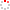#CodeStepByStep## assignGroups

Language/Type: C++ Graph collections

Suppose that a course instructor wants to assign discussion groups for in-class activities. To help everyone meet as many new people as possible, we want to make sure that no student is in a group with any of their friends. We can cast this as a graph problem where each student is a vertex and an edge exists between two students if they are friends (we consider friendship to be a mutual relationship, so the graph is undirected).

Write a function named `assignGroups` that will assign a group number to each student so that no two vertexes that share an edge have the same group number. What makes this problem tricky is that you will have a limited number of different groups to work with. Your function will return a Boolean value indicating whether or not it was possible to assign the groups successfully.

Your function accepts a reference to a `BasicGraph` and an integer nGroups. Group numbers should be assigned as integers in the range 1 to nGroups, inclusive. If you find a valid group assignment scheme, print it out to cout, using the exact format shown below (vertex name and group number separated by a space, each vertex on its own line), and return `true`. If it is not possible to separate all students from their friends using at most nGroups different groups, then print `"Group assignment impossible!"`, and return `false`. If there is more than one valid way of assigning groups, print only one valid way. If the value of nGroups. passed is 0 or negative, immediately return `false`.

Graph 1:Graph 1, nGroups = 3, expected output:

```A 1
B 2
C 1
D 2
E 3
F 1
G 2
```
Graph 2:Graph 2, nGroups = 2, expected output:

```Group assignment impossible!
```

There are several ways to solve this problem. One is to consider each vertex one at a time, trying each different group for it, then continuing on to see if the rest of the vertexes can be successfully grouped given the groups you have already assigned. If grouping the rest of the graph given your selection can't be done, you'll have to go back and try a different arrangement, until you find a valid one or prove that it can't be done. (Our description of this algorithm is intentionally somewhat vague because we want you to think about the details of how to implement it yourself.) Some of the algorithms you have seen, like Eight Queens and Dice Sum, "pruned" their searches by not conducting explorations that were known to fail. You should do the same on this problem. For example, if at any point in your algorithm you give two neighboring vertexes the same number, the graph's state is invalid and you should not explore that path any further.

Assumptions: You may assume that the graph's state is valid and that no two vertices have the same name. You may also assume that the graph is connected (that there exists a path from every vertex to every other vertex) and that it is undirected, in the sense that if there is an edge from vertex V1 to V2, there is also an edge from V2 to V1. You may assume that the graph contains no self-edges (e.g. from V1 to V1).

Constraints: You may define private helper functions if so desired, and you may construct auxiliary collections as needed to solve this problem. You should not modify the structure of the graph (e.g., add or remove vertexes or edges), but you may wish to modify the state variables inside individual vertexes/edges (e.g., cost, visited, color, etc.).

Type your C++ solution code here:

This is a function exercise. Write a C++ function as described. Do not write a complete program; just the function(s) above.